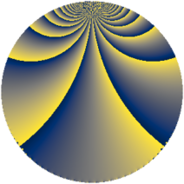# Properties

 Label 2736.3.cqLevel $2736$ Weight $3$ Character orbit 2736.cq Rep. character $\chi_{2736}(1265,\cdot)$ Character field $\Q(\zeta_{6})$ Dimension $476$ Sturm bound $1440$

# Related objects

## Defining parameters

 Level: $$N$$ $$=$$ $$2736 = 2^{4} \cdot 3^{2} \cdot 19$$ Weight: $$k$$ $$=$$ $$3$$ Character orbit: $$[\chi]$$ $$=$$ 2736.cq (of order $$6$$ and degree $$2$$) Character conductor: $$\operatorname{cond}(\chi)$$ $$=$$ $$171$$ Character field: $$\Q(\zeta_{6})$$ Sturm bound: $$1440$$

## Dimensions

The following table gives the dimensions of various subspaces of $$M_{3}(2736, [\chi])$$.

Total New Old
Modular forms 1944 484 1460
Cusp forms 1896 476 1420
Eisenstein series 48 8 40

## Trace form

 $$476q + q^{3} - 3q^{5} + 2q^{7} + 7q^{9} + O(q^{10})$$ $$476q + q^{3} - 3q^{5} + 2q^{7} + 7q^{9} + 6q^{11} - 2q^{13} + 19q^{15} + 72q^{17} + 4q^{19} - 10q^{21} + 1151q^{25} + 4q^{27} - 3q^{29} + 26q^{31} - 40q^{33} + 75q^{35} - 8q^{37} + 13q^{39} - 39q^{41} + 98q^{43} + 21q^{45} + 3q^{47} - 1584q^{49} + 19q^{51} + 27q^{55} + 71q^{57} + 3q^{59} + q^{61} - 169q^{63} + 138q^{65} + 2q^{67} + 5q^{69} - 14q^{73} + 356q^{75} - 6q^{77} + 2q^{79} - 217q^{81} + 6q^{83} - 102q^{85} - 117q^{87} + 49q^{91} + 17q^{93} + 651q^{95} - 122q^{97} + 99q^{99} + O(q^{100})$$

## Decomposition of $$S_{3}^{\mathrm{new}}(2736, [\chi])$$ into newform subspaces

The newforms in this space have not yet been added to the LMFDB.

## Decomposition of $$S_{3}^{\mathrm{old}}(2736, [\chi])$$ into lower level spaces

$$S_{3}^{\mathrm{old}}(2736, [\chi]) \cong$$ $$S_{3}^{\mathrm{new}}(171, [\chi])$$$$^{\oplus 5}$$$$\oplus$$$$S_{3}^{\mathrm{new}}(342, [\chi])$$$$^{\oplus 4}$$$$\oplus$$$$S_{3}^{\mathrm{new}}(684, [\chi])$$$$^{\oplus 3}$$$$\oplus$$$$S_{3}^{\mathrm{new}}(1368, [\chi])$$$$^{\oplus 2}$$